Custom

# How to Use Timers on the ATmega168June 19, 2018 by Robin Mitchell
Share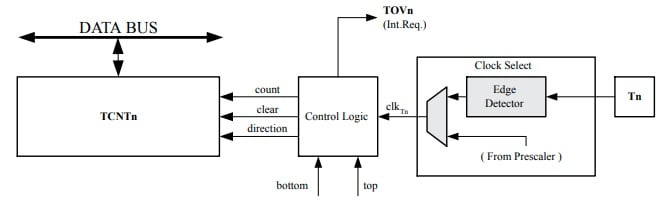Learn how to use Timer 0 on the ATmega168 in both normal and compare modes to create timing events for your projects!

## Software

 1 Atmel Studio 7 1 WinAVR

## Hardware

 1 USBasp 1 Atmega168 1 680R Resistor (R2) 1 5.6K Resistor (R1) 2 20pF Capacitor (C1, C2) 2 100nF Capacitor (C4, C5) 1 100uF Capacitor (C3) 1 7805 Regulator (U2 – Optional if not using USBasp power)

So far we have learned how to program an ATmega device, use basic bit manipulation, use I/O, and take analog measurements via the ADC. In this tutorial, we will learn how to use Timer 0 on the ATmega168 in two different modes: normal mode and compare mode.

### Schematic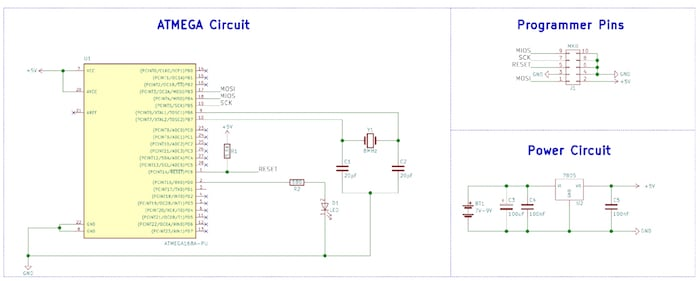### Why Use A Timer?

Most microcontroller projects will require the use of careful timing for events, including multi-tasking, bit banging protocols, measurement, and much more. These timing events can be done in software via the use of incrementing a counter on each iteration of a loop. However, this wastes CPU resources that could be used to do something else, and such use of loops can be difficult to time correctly. This is why timers were introduced to microcontrollers. Now they are so common that it is rare to find a controller without one.

Most ATmega devices have at least one timer, and the ATmega168 has three timers. So, in this tutorial, we will look at timer 0 and how we can use it for timing events when used in two different modes: normal and compare.

### Timer 0

Timer 0 is a generic 8-bit timer with some rather powerful features, including compare modes, fast-PWM generation, and waveform generation capabilities. While Timer 0 may look complicated, it is in fact rather simple to use, so long as you understand the basics behind how it works.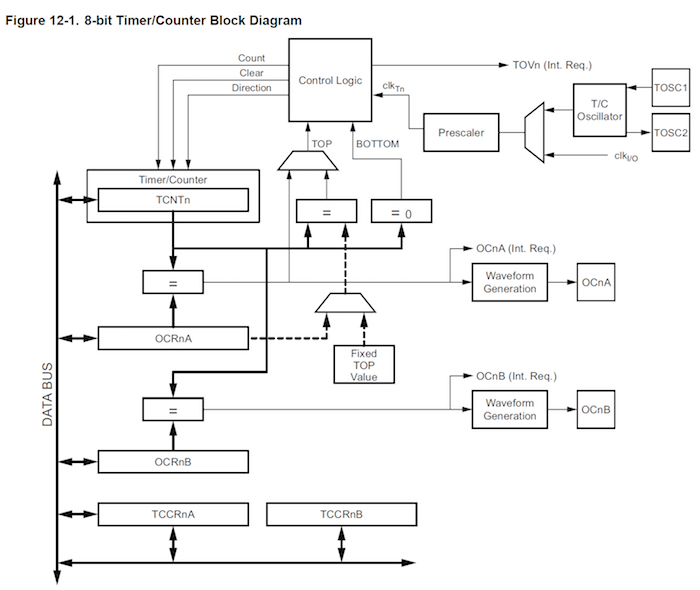Timer 0 peripheral layout

Firstly, timers on the AVR are almost identical to a simple up counter consisting of a bunch of flip-flops in a chain. Each time the timer is clocked it will increment a counter register that keeps track of the current timer value.

When the timer reaches its maximum value and is then clocked (timer0 is an 8-bit counter which means it has a maximum value of 255), the timer wraps around back to 0 and a timer overflow bit is set. This bit can be used to see if the counter has overflowed and is useful in situations to determine if a particular piece of code has stalled or is not responding.

Timers can usually be clocked from different sources, including internal clock sources and external I/O pins. This means that an external circuit could provide a square wave that increments the timer, or the microcontroller itself could increment the timer (this is usually used as the clock source).

Some timers (like timer 0) have compare units, which allow the timer to trigger an interrupt when the timer is equal to a certain value. This is incredibly useful when the microcontroller needs to do events that execute when a specific amount of time has passed.

One such example would involve the creation of video signals when synchronizing pulses need to be sent once every 64uS (PAL). Other examples would include multi-tasking, where the microcontroller can switch to a different task once every millisecond. Such compare units can also be made to clear the timer once a match occurs so the user does not have to reset the timer themselves.

### Timer 0: Normal Mode

In normal mode, timer 0 will increment on each clock, and once the counter passes its highest value (255 since itâ€™s an 8-bit timer), the timer wraps round to a value of 0 and an overflow bit is set (TOV0 bitâ€“found in register TIFR0).

To set timer 0 to run in normal mode, the bits WGM02â€“WGM01 need to be set to 0 (note; WGM02 is found in TCCR0B, while bits WGM01 and WGM00 are found in register TCCR0A.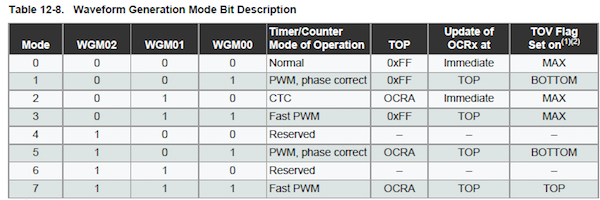Waveform bits found in register TCCR0A and TCCR0B

### Timer 0: CTC Mode

Clear Timer on Compare Match mode (CTC) is similar to normal mode, except when the timer reaches the value of register OCR0A, the timer is cleared (reset to a value of 0x00). This can be used to create timed events, including delay and interrupts without using software resource (all done in hardware).

When the timer equals the value of OCR0A, then OCF0A is set, which indicates that a match occurred between the timer and OCR0A. To configure timer 0 in CTC mode the bits WGM02â€“WMG00 need to be set to a value of 0x02.

### Timer 0 Clock Source

Timer 0 can be clocked from an external source (via the T0 pin), or from the internal I/O clock. Some of the I/O clock sources can be prescaled as shown in the table and the clock source selection bits are found in the TCCR0B register.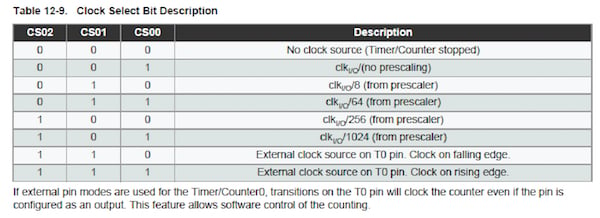Extract from the ATmega datasheet showing prescale options

### A Note on Interrupt Flags

It is important to note that AVR made it so that to clear a flag, you must write a logical one to the flag. This means that, for example, if you wanted to clear the overflow flag, you would write a 1 to that register INSTEAD of writing 0.

### Example 1: Normal Mode

This mode shows timer 0 being used in normal mode to turn an LED on and off every time the counter rolls over (over 255).

``````/*
* AVR Timer.c
*
* Created: 08/01/2018 13:16:36
* Author : RobinLaptop
*/

#define setBit(reg, bit) (reg = reg | (1 << bit))
#define clearBit(reg, bit) (reg = reg & ~(1 << bit))
#define toggleBit(reg, bit) (reg = reg ^ (1 << bit))
#define clearFlag(reg, bit) (reg = reg | (1 << bit))

#include <avr/io.h>

int main(void)
{
// Initialize Registers

clearBit(TCCR0A, WGM00);			// Configure WGM to be 0x00 for normal mode
clearBit(TCCR0A, WGM01);
clearBit(TCCR0B, WGM02);

setBit(TCCR0B, CS00);				// Configure clock source to be clock io at 1024 pre-scale
clearBit(TCCR0B, CS01);
setBit(TCCR0B, CS02);

DDRD = 0xFF;						// Make PORT D and output

while (1)
{
// Wait until the TOV0 bit is set
while(!(TIFR0 & (1 << TOV0)))
{

}

// Clear the overflow flag by writing a 1 to it. I know, thats dumb but that's how it is!
clearFlag(TIFR0, TOV0);

// Toggle the LED (PD0 , Pin 2)
PORTD = PORTD ^ (1 << PD0);
}
}``````

### Example 2: CTC Mode

This mode will toggle the LED when timer 0 is equal to the value of OCR0A. Once a match occurs, the timer will automatically reset itself and set the OCF0A flag.

``````/*
* Example 2 - CTC.c
*
* Created: 08/01/2018 13:43:06
* Author : RobinLaptop
*/

#define setBit(reg, bit) (reg = reg | (1 << bit))
#define clearBit(reg, bit) (reg = reg & ~(1 << bit))
#define toggleBit(reg, bit) (reg = reg ^ (1 << bit))
#define clearFlag(reg, bit) (reg = reg | (1 << bit))

#include <avr/io.h>

int main(void)
{
// Initialize Registers

clearBit(TCCR0A, WGM00);			// Configure WGM to be 0x00 for normal mode
setBit(TCCR0A, WGM01);
clearBit(TCCR0B, WGM02);

setBit(TCCR0B, CS00);				// Configure clock source to be clock io at 1024 pre-scale
clearBit(TCCR0B, CS01);
setBit(TCCR0B, CS02);

DDRD = 0xFF;						// Make PORT D and output

OCR0A = 0x7F;						// Reset the timer once the value of the timer reaches 127

while (1)
{
// Wait until the OCF0A bit is set
while(!(TIFR0 & (1 << OCF0A)))
{

}

// Clear the overflow flag by writing a 1 to it. I know, thats dumb but that's how it is!
clearFlag(TIFR0, OCF0A);

// Toggle the LED (PD0 , Pin 2)
PORTD = PORTD ^ (1 << PD0);
}
}``````

### Conclusion

This tutorial only touches on timers with timers being able to do much more. For example, these timers could have their interrupts enabled which would allow the microcontroller to run time-sensitive code as soon as the flag is set. Or, instead of doing a while loop to wait for the overflow flag to trigger, we could have executed other code, which would utilize the CPU more efficiently. Clearly, timers are very powerful and can make a massive difference to most projects!

Images courtesy of Microchip's ATmega 8-bit AVR Microcontroller datasheet (PDF).

### AuthorRobin Mitchell

Graduated from the University Of Warwick in Electronics with a BEng 2:1 and currently runs MitchElectronics.